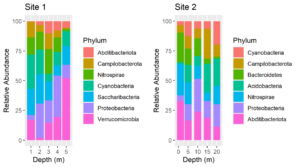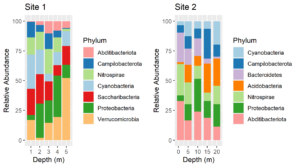## Using Named Colors with ggplot2

Consider these two plots:The colors assigned to the phyla are not the same in the two plots. We cannot make them so simply by faceting because there are unique phyla at each site and the sites were sampled at different depths.  In fact, they were plotted using different tibbles. Still, it would be much easier to interpret the plots if the same colors were assigned to the phyla that are common between the plots. We can do this by using a palette of named colors.

First, get a vector of the union of unique phyla for each plot and determine its length. The plots were made from two tibbles, `df1` and `df2`.

````unique.phyla <- union(unique(df1\$Phylum), unique(df2\$Phylum))````
length(unique.phyla)``````
 9``````

So while there are only 7 phyla in each plot, there are a total of 9 in the two plots. Next, get a vector of 9 colors and name the colors with the names of the phyla.

``````my.colors <- RColorBrewer::brewer.pal(n = length(unique.phyla),name = "Paired")
names(my.colors) <- unique.phyla
my.colors
Cyanobacteria  Campilobacterota  Nitrospirae Proteobacteria
"#A6CEE3"      "#1F78B4"         "#B2DF8A"       "#33A02C"
Abditibacteriota Saccharibacteria Verrucomicrobia Acidobacteria
"#FB9A99"        "#E31A1C"        "#FDBF6F"       "#FF7F00"
Bacteroidetes
"#CAB2D6"``````

Replot, using the named palette for the fill colors. The plots above were made using the same code as below except that the `scale_fill_manual` lines were omitted.

````plt1 <- ggplot(df1, aes(x=Depth, y=value, fill = Phylum)) +````
geom_col() +``````
ylab("Relative Abundance") +
``````   scale_fill_manual(values = my.colors, aesthetics = "fill") +
````    xlab("Depth (m)") +````
ggtitle("Site 1")
````plt2 <- ggplot(df2, aes(x=Depth, y=value, fill = Phylum)) +````
geom_col() +``````
scale_fill_manual(values = my.colors, aesthetics = "fill") +
````    ylab("Relative Abundance") +` ```xlab("Depth (m)") +
``````    gtitle("Site 2")
``````
cowplot::plot_grid(plt1, plt2)``````Voila! Now the color assignments are consistent between phyla. This approach can be generalized to solve the same problem with other types of plots.

## Compact Letter Displays

Compact letter displays (CLDs) are letters that show which treatment groups are not significantly different by some statistical test. It is often desirable to include CLDs on graphs. Here I show how to add them to a box plot created with ggplot2. Continue reading “Compact Letter Displays”

## Get ggplot plot panel limits

I have added a new function (`get_plot_limits`) to my package `QsRutils`.  It extracts the minimum and maximum X and Y values for a ggplot panel. This is useful in formatting ggplots. For example, you may wish to expand the panel to avoid text running out of the panel, or nudge text relative to some point. For an example, see my post on adding compact letter displays to box plots created with `ggplot2`.

## Rotatable 3D Plots

The R package vegan includes the function ordiplot for making ordination plots using R’s base graphics. Additionally vegan provides several functions for enhancing the plots with spiders, hulls, and ellipses. It is even possible to overlay an ordination plot with a cluster diagram. (See my package ggordiplots on GitHub for making the same kinds of plots with ggplot graphics.) Vegan’s functions for adding these layers begin with “ordi”: ordispider, ordihull, ordiellipse, ordicluster. Earlier this year I discovered the package vegan3d. It makes use of rgl graphics which means that the plots it generates can be scaled and rotated with the mouse. This is not just fun – it allows you to see how well separated treatment groups are in the ordination space.